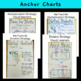# Multiplication & Division Word Problems - Anchor Charts, Mini Lesson & PracticeSubject
Resource Type
File Type

PDF

(2 MB|19 pages)
Product Rating
Standards
• Product Description
• StandardsNEW

Multiplication & Division Word Problems - I use this organizer format as my students start learning how to solve both multiplication and division word problems. It is extremely important for students to visualize the problem, pick out key words and figure out their operation, solve it correctly, and finally be able to explain how they got their answer. My students love using this organizer. This is also the 1st year that my students are excited about being able to explain what they did using math vocabulary.

Includes:

~Example of Word Problem Organizer for student notebooks

~Two Multiplication Anchor Charts (Partial Products & Base Ten Blocks)

~3 Word problems with the organizer (cut out to fit into journals for students to do during mini lesson)

~Center/Assessment (10 Multiplication Word Problems for practice)

~Two Division Anchor Charts (Partial Quotients & Area Model Division)

~4 Word problems with the organizer (cut out to fit into journals for students to do during mini lesson)

~Center/Assessment (10 Division Word Problems for practice)

Find whole-number quotients and remainders with up to four-digit dividends and one-digit divisors, using strategies based on place value, the properties of operations, and/or the relationship between multiplication and division. Illustrate and explain the calculation by using equations, rectangular arrays, and/or area models.
Multiply a whole number of up to four digits by a one-digit whole number, and multiply two two-digit numbers, using strategies based on place value and the properties of operations. Illustrate and explain the calculation by using equations, rectangular arrays, and/or area models.
Total Pages
19 pages
Not Included
Teaching Duration
N/A
Report this Resource to TpT
Reported resources will be reviewed by our team. Report this resource to let us know if this resource violates TpT’s content guidelines.